2. The Robertson Walker Metric

The only metric compatible with Hubble's findings and the Cosmological Principle is the Robertson Walker Metric (RWM) with the corresponding line element,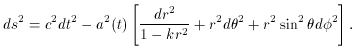(4)

For a brief explanation consider the following:

• For the metric to be homogeneous, isotropic, and obey the Weyl postulate, the metric must be the same in all directions and locations,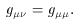• For a uniform expansion we must have a scale factor a(t) that is a function of time only.

• Allowance for any type of geometry (curvature) must be made. This is represented by the constant k, where k = 0, k = 1, and k = -1 corresponds to flat, spherical, and hyperbolic geometries, respectively.

There are a few subtleties that must be discussed. First, the r that appears in the line element (4) is not the radius of the universe. The r is a dimensionless, comoving coordinate that ranges from zero to one for k = 1. The measurable, physical distance is given by the RWM above. Choosing a frame common to two distinct points, one obtains,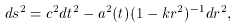for their separation. Where d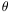and d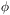are zero, because one has freedom to arrange the axis and ds2 represents their separation in spacetime. Thus, their spatial separation is found by considering spacelike hypersurfaces, that is dt2 = 0. Thus, their separation is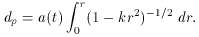Evidently for a k = 0 flat universe, the distance is simply,Thus, a(t) has units of length and depends on the geometry of the spacetime.

The next issue is that of curvature. The curvature of the universe is determined by the amount of energy and matter that is present. The space is one of constant curvature determined by the value of k. Because any arbitrary scaling of the line element (4) will not affect the sign of k, we have the following convention (11)

• k = 1 represents positive, spherical geometry
• k = 0 represents flat Minkowski space
• k = -1 represents negative, hyperbolic geometry

11 When the metric is invariant under multiplication by a scale factor, the metric is said to be conformally invariant. Back.